Anzeige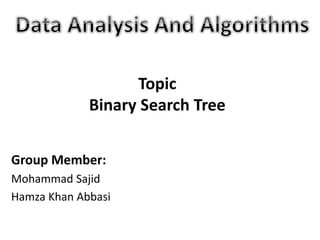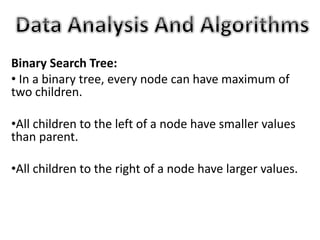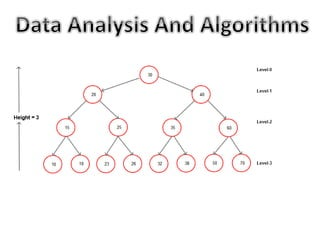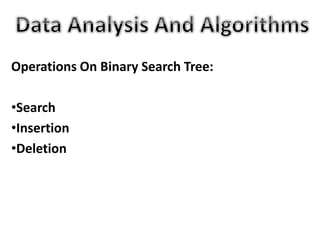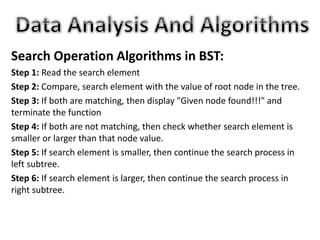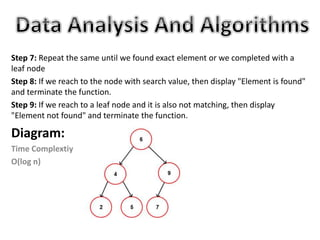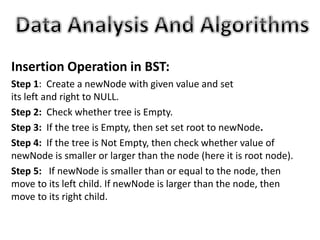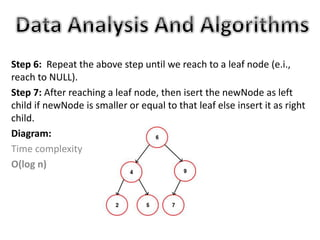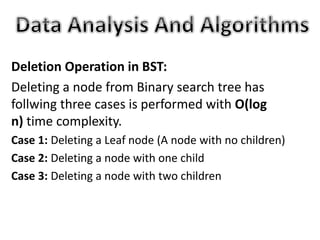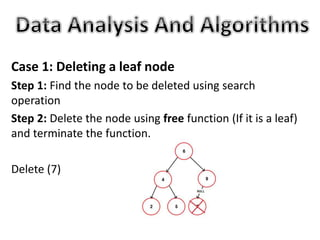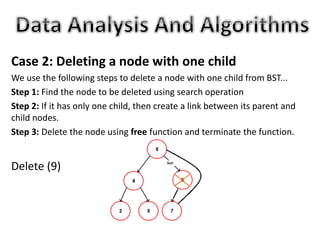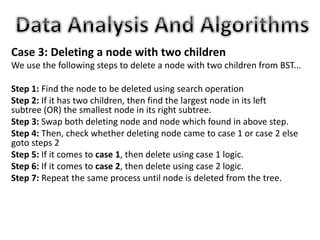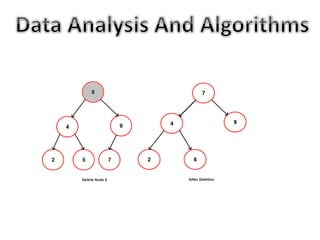1 von 14
Anzeige

### Binary Search Tree (BST)

1. Group Member: Mohammad Sajid Hamza Khan Abbasi Topic Binary Search Tree
2. Binary Search Tree: • In a binary tree, every node can have maximum of two children. •All children to the left of a node have smaller values than parent. •All children to the right of a node have larger values.
3. Operations On Binary Search Tree: •Search •Insertion •Deletion
4. Search Operation Algorithms in BST: Step 1: Read the search element Step 2: Compare, search element with the value of root node in the tree. Step 3: If both are matching, then display "Given node found!!!" and terminate the function Step 4: If both are not matching, then check whether search element is smaller or larger than that node value. Step 5: If search element is smaller, then continue the search process in left subtree. Step 6: If search element is larger, then continue the search process in right subtree.
5. Step 7: Repeat the same until we found exact element or we completed with a leaf node Step 8: If we reach to the node with search value, then display "Element is found" and terminate the function. Step 9: If we reach to a leaf node and it is also not matching, then display "Element not found" and terminate the function. Diagram: Time Complextiy O(log n)
6. Insertion Operation in BST: Step 1: Create a newNode with given value and set its left and right to NULL. Step 2: Check whether tree is Empty. Step 3: If the tree is Empty, then set set root to newNode. Step 4: If the tree is Not Empty, then check whether value of newNode is smaller or larger than the node (here it is root node). Step 5: If newNode is smaller than or equal to the node, then move to its left child. If newNode is larger than the node, then move to its right child.
7. Step 6: Repeat the above step until we reach to a leaf node (e.i., reach to NULL). Step 7: After reaching a leaf node, then isert the newNode as left child if newNode is smaller or equal to that leaf else insert it as right child. Diagram: Time complexity O(log n)
8. Deletion Operation in BST: Deleting a node from Binary search tree has follwing three cases is performed with O(log n) time complexity. Case 1: Deleting a Leaf node (A node with no children) Case 2: Deleting a node with one child Case 3: Deleting a node with two children
9. Case 1: Deleting a leaf node Step 1: Find the node to be deleted using search operation Step 2: Delete the node using free function (If it is a leaf) and terminate the function. Delete (7)
10. Case 2: Deleting a node with one child We use the following steps to delete a node with one child from BST... Step 1: Find the node to be deleted using search operation Step 2: If it has only one child, then create a link between its parent and child nodes. Step 3: Delete the node using free function and terminate the function. Delete (9)
11. Case 3: Deleting a node with two children We use the following steps to delete a node with two children from BST... Step 1: Find the node to be deleted using search operation Step 2: If it has two children, then find the largest node in its left subtree (OR) the smallest node in its right subtree. Step 3: Swap both deleting node and node which found in above step. Step 4: Then, check whether deleting node came to case 1 or case 2 else goto steps 2 Step 5: If it comes to case 1, then delete using case 1 logic. Step 6: If it comes to case 2, then delete using case 2 logic. Step 7: Repeat the same process until node is deleted from the tree.
Anzeige